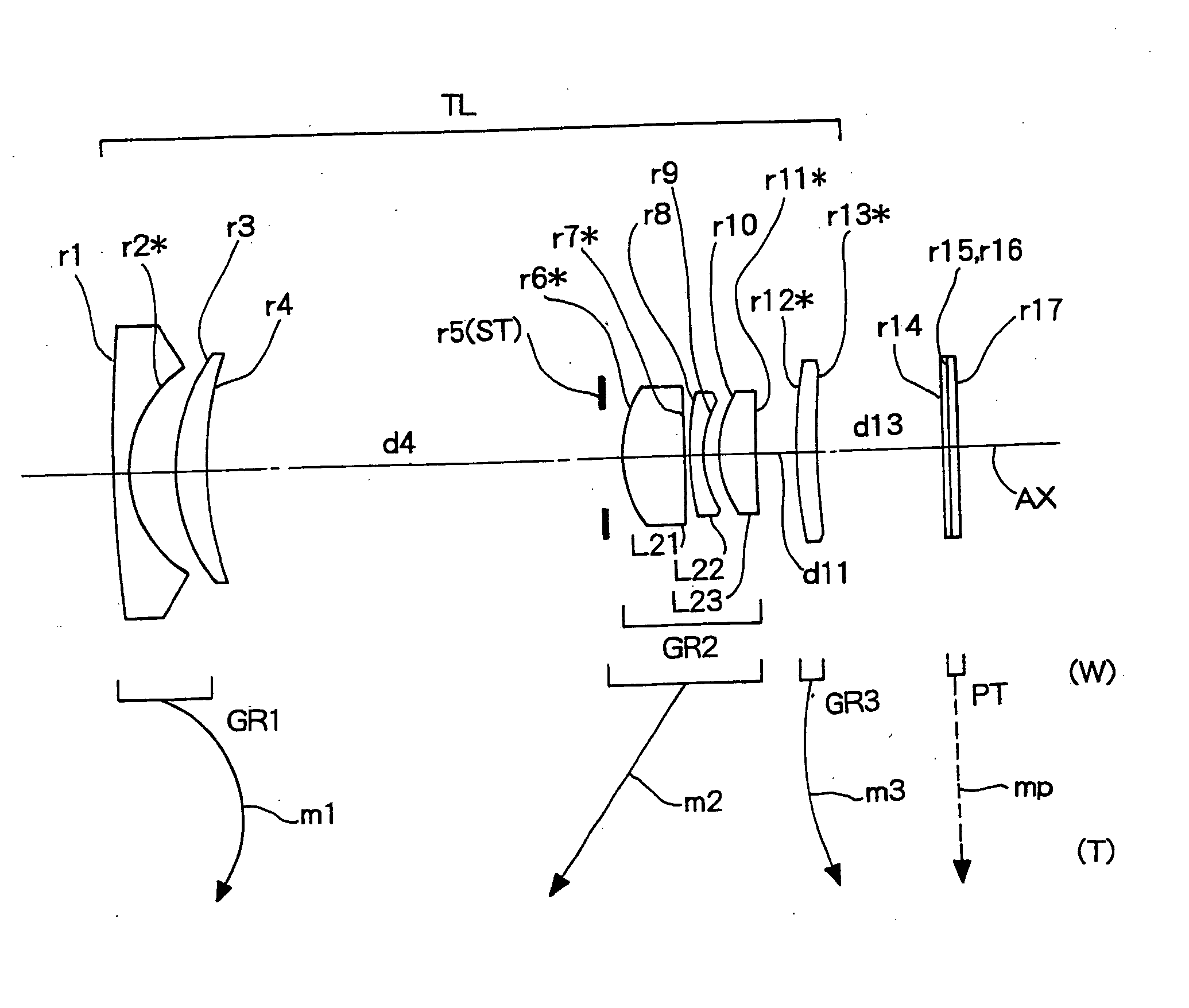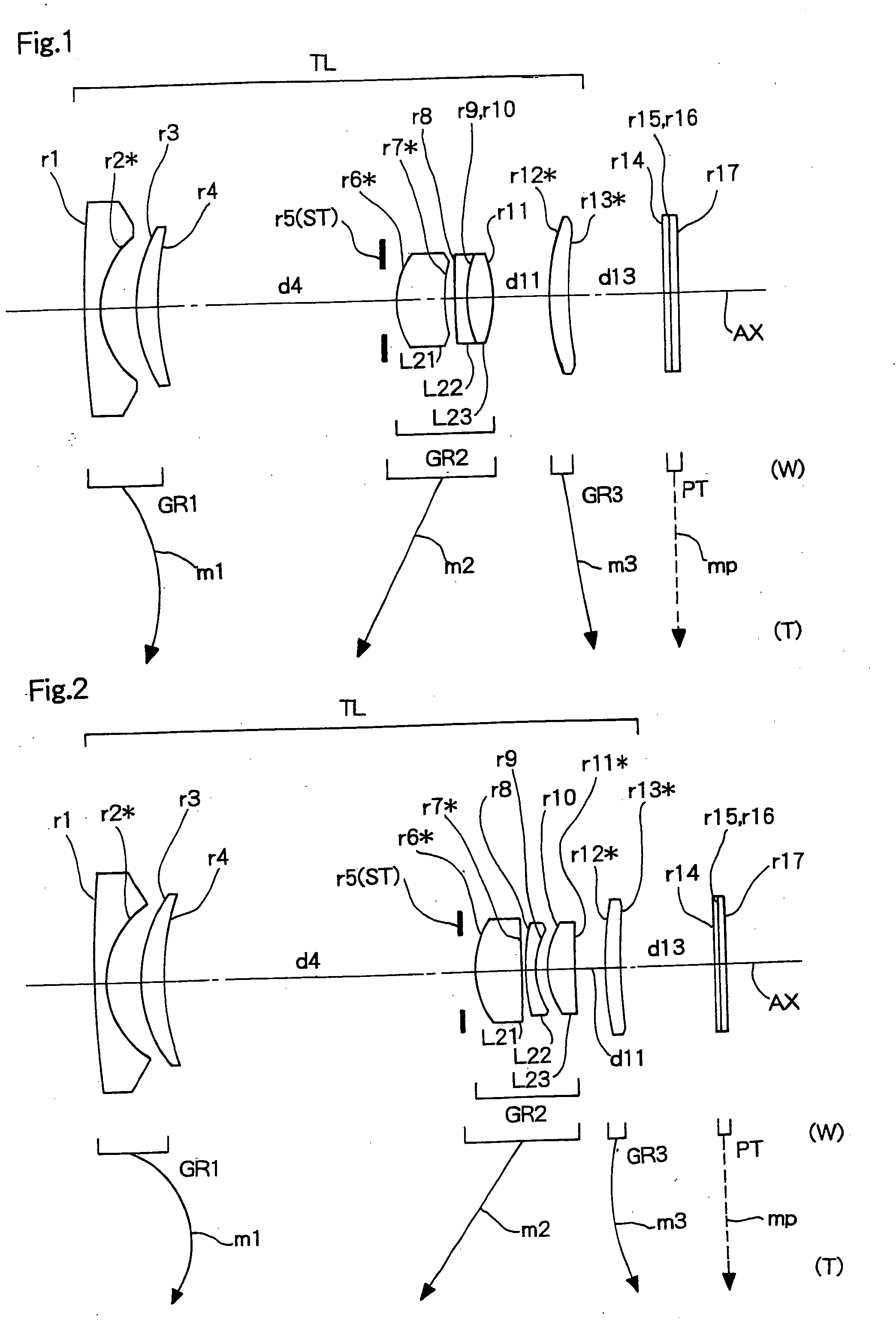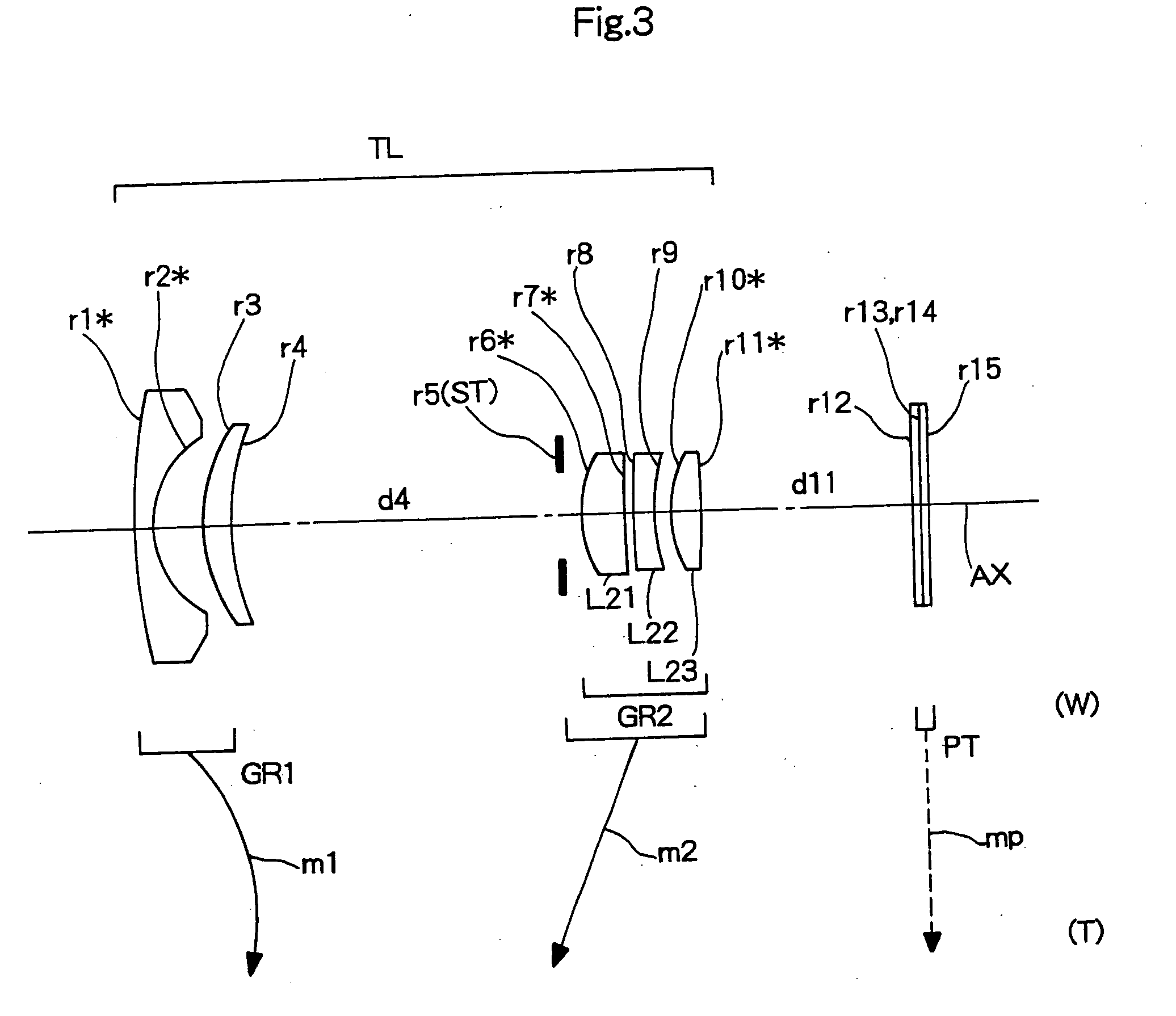# Image forming device

Inactive Publication Date: 2006-02-02
KONICA MINOLTA PHOTO IMAGING
3 Cites 28 Cited by

## AI-Extracted Technical Summary

### Problems solved by technology

However, in conventional zoom lens systems like that proposed in the above-mentioned patent application publication, it is d...
the structure of the environmentally friendly knitted fabric provided by the present invention; figure 2 Flow chart of the yarn wrapping machine for environmentally friendly knitted fabrics and storage devices; image 3 Is the parameter map of the yarn covering machine
View more

### Method used

 As described above, in all of these embodiments, the second lens unit GR2 comprises three lens elements of, from the object side, the positive meniscus lens element L21 convex to the object side, the negative lens element L22 and the positive lens element L23. By forming the second lens unit of three lens elements of, from the object side, a positive meniscus lens element convex to the object side, a negative lens element and a positive lens element like in each embodiment in a zoom lens system including from the object side a first lens unit having negative optical power and a second lens unit having positive optical power as movable units, a compact zoom lens system can be realized in which the number of lens elements is small and the movement mechanism is comparatively simple. Moreover, by forming the second lens unit of three single lens elements of a meniscus lens element convex to the object side, a negative lens element and a positive lens element like the second and third embodiments and forming the second lens unit of a meniscus lens element convex to the object side and a doublet lens having a negative lens element and a positive lens element like the first embodiment, aberration correction can be excellently performed.
 The condition (1) defines a preferable condition range with respect to a shape factor of the most object side lens element (the positive meniscus lens element L21 convex to the object side in each embodiment) in the second lens unit. When the lower limit of the condition (1) is exceeded, the most object side lens element is a bi-convex lens element, and the error sensitivity of the distance between the most object side lens element and the succeeding lens element increases. To reduce the error sensitivity, it cannot be helped that the overall length is increased.
 Conversely, when the upper limit of the condition (1) is exceeded, aberration correction, particularly, correction of spherical aberration at the telephoto end is difficult. Consequently, it is necessary to increase the overall length or add a lens element. By adopting the three-lens-unit construction to the second lens unit and satisfying the condition (1), compactness is achieved while sufficient optical performance is ensured, and the error sensitivity of the lens elements in the second lens unit can be reduced. Consequently, an image forming device can be realized that has a zoom lens system being easy to manufacture although being small in size and high in performance. The “error sensitivity” referred to here is the error sensitivity of the spherical aberration by the axial thickness of the lens elements and the distance between the lens elements.
 By satisfying the condition (1) in each embodiment, an image forming device LU having an easy-to-manufacture zoom lens system TL can be realized by reducing the error sensitivity in the second lens unit GR2 while compactness and high optical performance are ensured in a zoom lens system TL comprising three lens units of negative, positive, positive configuration or two lens units of negativ...
the structure of the environmentally friendly knitted fabric provided by the present invention; figure 2 Flow chart of the yarn wrapping machine for environmentally friendly knitted fabrics and storage devices; image 3 Is the parameter map of the yarn covering machine
View more

### Benefits of technology

 The present invention is made in view of such circumstances, and an object thereof is to provide an image form...
the structure of the environmentally friendly knitted fabric provided by the present invention; figure 2 Flow chart of the yarn wrapping machine for environmentally friendly knitted fabrics and storage devices; image 3 Is the parameter map of the yarn covering machine
View more

## Abstract

An image forming device is provided with: a zoom lens system TL that performs magnification varying by changing the distance between the lens units; and an image sensor SR that converts an optical image formed by the zoom lens system TL into an electric signal. The zoom lens system TL includes at least a negative first lens unit GR1 and a positive second lens unit GR2, and at least the first lens unit GR1 and the second lens unit GR2 move in zooming from the wide angle end (W) to the telephoto end (T). The second lens unit GR2 includes three lens elements of, from the object side, a positive meniscus lens element L21 convex to the object side, a negative lens element L22 and a positive lens element L23, and the following condition is satisfied:
−1.0<(CR1−CR2)/(CR1+CR2)<0.0
(CR1 is the front radius of curvature of the most object side lens element L21 in the second lens unit GR2, and CR2 is the rear radius of curvature of the most object side lens element L21 in the second lens unit GR2).

Application Domain

Technology Topic

## Image

•••## Examples

• Experimental program(3)

### Example

EXAMPLE 1

f=5.50−9.29−15.68
FNO.=3.05−3.73−4.90 [Radius of Curvature] [Axial Distance] [Refractive Index(Nd)] [Abbe Number(νd)] r1 = 80.640 d1 = 1.000 N1 = 1.76743 ν1 = 49.48 r2* = 5.300 d2 = 2.804 r3 = 10.767 d3 = 1.723 N2 = 1.84666 ν2 = 23.78 r4 = 23.023 d4 = 16.761-7.087-1.498 r5 = INF(ST) d5 = 1.000 r6* = 6.000 d6 = 3.367 N3 = 1.53048 ν3 = 55.72 (L21(+)) r7* = 7.652 d7 = 0.832 r8 = 45.902 d8 = 0.800 N4 = 1.80518 ν4 = 25.46 (L22(−)) r9 = 8.401 d9 = 0.005 N5 = 1.51400 ν5 = 42.83 r10 = 8.401 d10 = 1.948 N6 = 1.58913 ν6 = 61.25 (L23(+)) r11 = −8.598 d11 = 4.079-10.312-20.865 r12* = 15.195 d12 = 1.451 N7 = 1.53048 ν7 = 55.72 (GR3(+)) r13* = 30.000 d13 = 7.161-5.987-3.248 r14 = INF d14 = 0.500 N8 = 1.51680 ν8 = 64.20 r15 = INF d15 = 0.000 r16 = INF d16 = 0.500 N9 = 1.51680 ν9 64.20 r17 = INF [Aspherical Coefficient] r2* ε = 0.3020 A4 = −0.64908782E−04 A6 = 0.46698117E−05 A8 = −0.42637540E−06 A10 = 0.14545351E−07 A12 = −0.18588005E−09 r6* ε = 1.0000 A4 = −0.49251260E−03 A6 = −0.82311406E−05 A8 = −0.51450757E−06 r7* ε = 1.0000 A4 = 0.29787285E−03 A6 = −0.86070840E−05 A8 = −0.59337948E−06 r12* ε = 1.0000 A4 = 0.52842825E−03 A6 = −0.12107356E−04 A8 = −0.37289244E−06 r13* ε = 1.0000 A4 = 0.69778302E−03 A6 = −0.88594967E−05 A8 = −0.76728843E−06

### Example

EXAMPLE 2

f=5.80−9.53−21.38
FNO.=2.88−3.44−5.00 [Radius of Curvature] [Axial Distance] [Refractive Index(Nd)] [Abbe Number(νd)] r1 = 67.905 d1 = 1.000 N1 = 1.76743 ν1 = 49.48 r2* = 6.433 d2 = 3.141 r3 = 11.824 d3 = 1.796 N2 = 1.84666 ν2 = 23.78 r4 = 22.284 d4 = 25.605-12.044-1.680 r5 = INF(ST) d5 = 1.000 r6* = 6.093 d6 = 4.071 N3 = 1.53048 ν3 = 55.72 (L21(+)) r7* = 50.000 d7 = 0.360 r8 = 20.955 d8 = 0.800 N4 = 1.80518 ν4 = 25.46 (L22(−)) r9 = 5.916 d9 = 1.107 r10 = 7.246 d10 = 2.325 N5 = 1.53048 ν5 = 55.72 (L23(+)) r11* = −71.675 d11 = 2.500-5.347-22.792 r12* = 22.312 d12 = 1.447 N6 = 1.53048 ν6 = 55.72 (GR3(+)) r13* = 72.018 d13 = 8.074-9.180-5.470 r14 = INF d14 = 0.500 N7 = 1.51680 ν7 = 64.20 r15 = INF d15 = 0.000 r16 = INF d16 = 0.500 N8 = 1.51680 ν8 = 64.20 r17 = INF [Aspherical Coefficient] r2* ε = 0.2912 A4 = 0.37573117E−04 A6 = 0.62712310E−06 A8 = −0.33626912E−07 A10 = 0.83547899E−09 A12 = −0.81452832E−11 r6* ε = 1.0000 A4 = −0.38219846E−03 A6 = −0.79507576E−05 A8 = −0.41873850E−06 r7* ε = 1.0000 A4 = −0.40552524E−03 A6 = −0.18911411E−04 A8 = 0.34838293E−06 r11* ε = 1.0000 A4 = 0.62163745E−03 A6 = 0.20427617E−04 A8 = −0.83798643E−07 r12* ε = 1.0000 A4 = −0.13311619E−03 A6 = 0.77951826E−05 A8 = −0.21320862E−06 r13* ε = 1.0000 A4 = −0.95450693E−04 A6 = 0.46213242E−05 A8 = −0.13961099E−06

### Example

EXAMPLE 3

f=5.54−9.35−15.79
FNO.=3.30−3.89−4.90
 [Radius of Curvature] [Axial Distance] [Refractive Index(Nd)] [Abbe Number(νd)] r1* = 72.995 d1 = 1.000 N1 = 1.76743 ν1 = 49.48 r2* = 5.300 d2 = 2.832 r3 = 9.000 d3 = 1.737 N2 = 1.84666 ν2 = 23.78 r4 = 14.852 d4 = 19.090-8.228-1.791 r5 = INF(ST) d5 = 1.000 r6* = 6.000 d6 = 2.422 N3 = 1.53048 ν3 = 55.72 (L21(+)) r7* = 20.000 d7 = 0.574 r8 = 49.627 d8 = 1.200 N4 = 1.84666 ν4 = 23.78 (L22(−)) r9 = 10.000 d9 = 1.000 r10* = 8.676 d10 = 1.797 N5 = 1.48749 ν5 = 70.44 (L23(+)) r11* = −13.433 d11 = 12.277-16.133-22.644 r12 = INF d12 = 0.500 N6 = 1.51680 ν6 = 64.20 r13 = INF d13 = 0.000 r14 = INF d14 = 0.500 N7 = 1.51680 ν7 = 64.20 r15 = INF [Aspherical Coefficient] r1* ε = 1.0000 A4 = 0.48597091E−03 A6 = −0.17926702E−04 A8 = 0.29793659E−06 A10 = −0.24929599E−08 A12 = 0.13042277E−10 r2* ε = 0.7167 A4 = 0.43141973E−03 A6 = −0.16407899E−04 A8 = −0.73786990E−06 A10 = 0.16896544E−07 A12 = −0.10290956E−09 r6* ε = 1.0000 A4 = −0.49852456E−03 A6 = 0.63781505E−06 A8 = −0.32900976E−05 A10 = −0.20332202E−06 A12 = 0.15824618E−07 r7* ε = 1.0000 A4 = −0.27120560E−03 A6 = 0.38608122E−05 A8 = −0.78018741E−05 A10 = −0.50413392E−06 A12 = 0.58559737E−07 r10* ε = 1.0000 A4 = 0.80071779E−03 A6 = 0.40727901E−04 A8 = 0.65174734E−05 A10 = −0.89999954E−06 A12 = 0.38805654E−07 r11* ε = 1.0000 A4 = 0.15912591E−02 A6 = 0.60281911E−04 A8 = 0.97926390E−05 A10 = −0.49341625E−06 A12 = 0.34023824E−08
 TABLE Condition 1 (CR1 − CR2)/(CR1 + CR2) −0.121 −0.783 −0.538 Condition 2 Np 1.530(L21) 1.530(L21) 1.530(L21) Condition 3 PW · (N′− N) · −0.002(r6*) −0.006(r6*) −0.003(r6*) d{x(y) − X0(y)}/dy Condition 4 f1/√(fw · ft) −1.381 −1.390 −1.292 Condition 5 TLw/fw/Z 2.871 2.573 2.978
 The above-described image forming device is an image forming device provided with: a zoom lens system comprising a plurality of lens units and performing magnification varying by changing the distance between the lens units; and an image sensor that converts an optical image formed by the zoom lens system into an electric signal, the zoom lens system comprises at least a first lens unit having negative optical power and a second lens unit having positive optical power from the object side, at least the first lens unit and the second lens unit move in zooming from the wide angle end to the telephoto end, the second lens unit comprises three lens elements of, from the object side, a positive meniscus lens element convex to the object side, a negative lens element and a positive lens element, and the following condition (1) is satisfied:
1.0 CR1−CR2)/(CR1+CR2)<0.0  (1)
where CR1 is the front radius of curvature of the most object side lens element in the second lens unit, and CR2 is the rear radius of curvature of the most object side lens element in the second lens unit.
 Moreover, in the above-described structure, in the image forming device, at least one positive lens element in the second lens unit satisfies the following condition (2):
1.4 1.7  (2)
where Np is the refractive index of the positive lens element in the second lens unit.
 Moreover, in the above-described structures, the image forming device satisfies the following condition (4):
−2.5 /√(fw·ft)<−0.7  (4)
where fl is the focal length of the first lens unit, fw is the overall focal length of the zoom lens system at the wide angle end, and ft is the overall focal length of the zoom lens system at the telephoto end.
 Moreover, in the above-described structures, in the image forming device, the zoom lens system comprises three lens units of, from the object side, a first lens unit having negative optical power, a second lens unit having positive optical power and a third lens unit having positive optical power, and the third lens unit comprises one positive lens element.
 Moreover, in the above-described structures, the image forming device satisfies the following condition (5):
1.5 3.5  (5)
where TLw is the optical overall length (the length from the most object side surface of the zoom lens system to the image surface) at the wide angle end, fw is the overall focal length of the zoom lens system at the wide angle end, and Z is the zoom ratio.
 Moreover, various cameras can be formed by use of the image forming device having the above-described structures.
 Although the present invention has been fully described by way of examples with reference to the accompanying drawings, it is to be noted that various in the art. Therefore, unless otherwise such changes and modifications depart from the scope of the present invention, they should be construed as being included therein.
the structure of the environmentally friendly knitted fabric provided by the present invention; figure 2 Flow chart of the yarn wrapping machine for environmentally friendly knitted fabrics and storage devices; image 3 Is the parameter map of the yarn covering machine

## PUM## Description & Claims & Application Information

We can also present the details of the Description, Claims and Application information to help users get a comprehensive understanding of the technical details of the patent, such as background art, summary of invention, brief description of drawings, description of embodiments, and other original content. On the other hand, users can also determine the specific scope of protection of the technology through the list of claims; as well as understand the changes in the life cycle of the technology with the presentation of the patent timeline. Login to view more.
the structure of the environmentally friendly knitted fabric provided by the present invention; figure 2 Flow chart of the yarn wrapping machine for environmentally friendly knitted fabrics and storage devices; image 3 Is the parameter map of the yarn covering machine

## Semiconductor device with alternating conductivity type layer and method of manufacturing the same

Owner:FUJI ELECTRIC CO LTD

## Soundproofing assembly for an automobile, and associated wall element

Owner:FAURECIA AUTOMOTIVE IND

## Connecting structure for hose with corrugated metal tube

Owner:TOKAI RUBBER IND LTD

## Voltage controlled clock synthesizer

Owner:SKYWORKS SOLUTIONS INC

## Integrated cup insert jounce bumper

Owner:VIBRACOUSTIC NORTH AMERICA

## Classification and recommendation of technical efficacy words

• Small size
• Easily manufacture

## Surgical stapling device

Owner:TYCO HEALTHCARE GRP LP

## Microfabricated elastomeric valve and pump systems

InactiveUS6929030B2High speedSmall sizeFixed microstructural devicesVolume/mass flow measurementEngineeringPlanar substrate
Owner:CALIFORNIA INST OF TECH

## Microfabricated elastomeric valve and pump systems

InactiveUS7144616B1High speedSmall sizeEngine diaphragmsFixed microstructural devicesPlanar substrateMechanical engineering
Owner:CALIFORNIA INST OF TECH

## RFID antenna

Owner:TRANSPACIFIC TECH

## Rectenna

Owner:IHI AEROSPACE CO LTD
Who we serve
• R&D Engineer
• R&D Manager
• IP Professional
Why Eureka